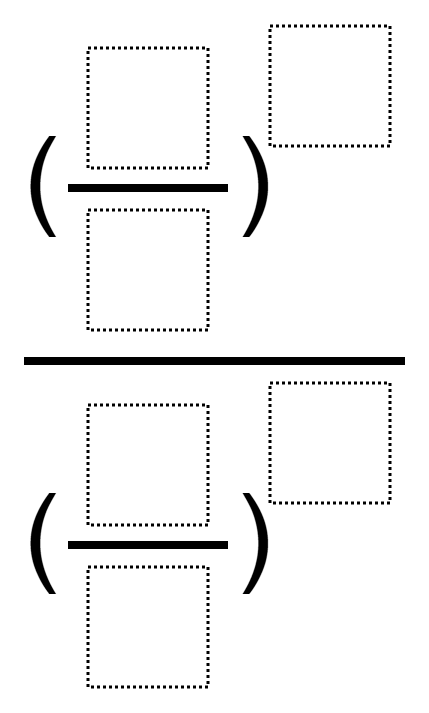Home > Grade 8 > Expressions & Equations > Simplifying Exponential Expressions

# Simplifying Exponential Expressions

Directions: Fill in the empty boxes with whole numbers 1 through 10, using each number at most once, so that the result is closest to 1.### Hint

What does closest to 1 mean? What does this tell you about the fraction in the numerator and the fraction in the denominator?

There are several answers that will give a result of 1, such as ((4/9)^5)/((2/3)^10).

If we are looking for an answer that is closest but not equal to 1, I’m not sure what the answer is!

Source: Daniel Luevanos

## Square Root Expression

Directions: Use the digits 1 to 9, at most one time each, to fill in …

1.2.